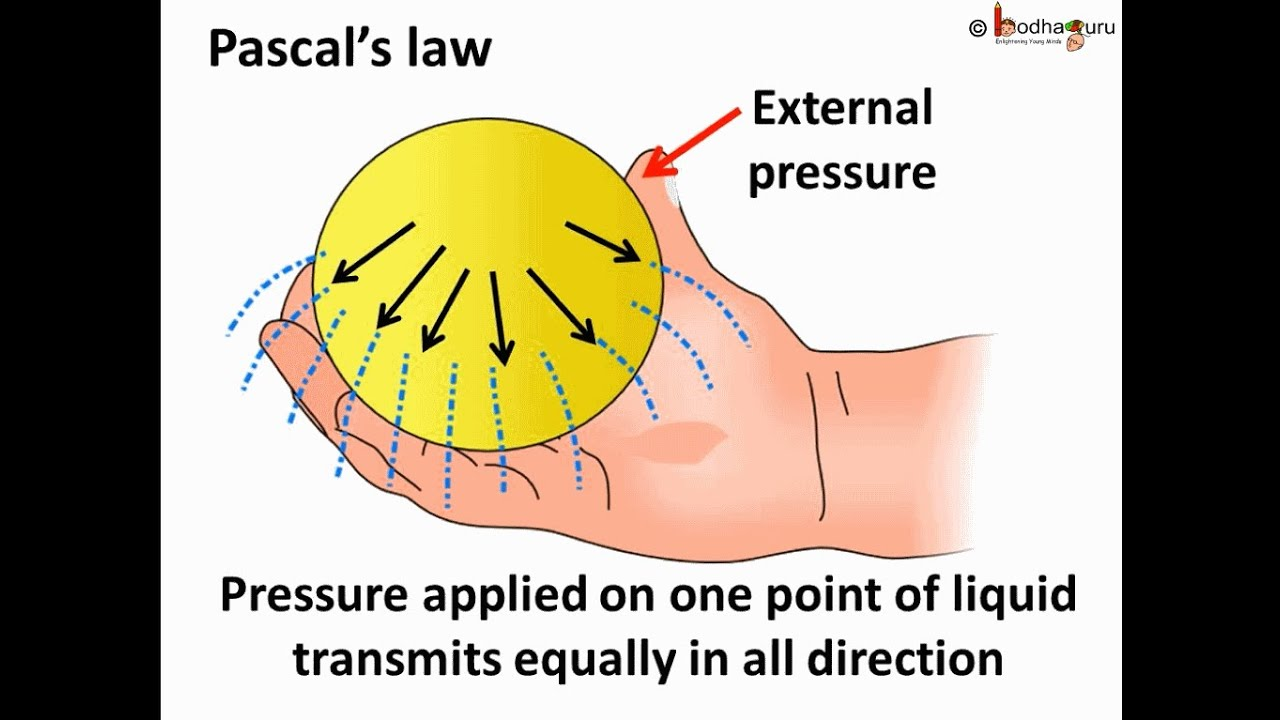Notes on Pascal's Law | Grade 10 > Science > Pressure | KULLABS.COM

Notes, Exercises, Videos, Tests and Things to Remember on Pascal's Law

Please scroll down to get to the study materials.

• Note
• Things to remember
• Videos
• Exercise
• Quiz

Pascal's law states that "Pressure exerted by liquid in an enclose vessel is transmitted equally". This law was formulated by Blaise Pascal who was born in 1623 in France. This law is applicable for the gases also. The verification of Pascal's law can be done with this simple experiment:Take a plastic (or a balloon) and poke small holes (carefully) in the plastic. Fill the plastic with water and seal the top big opening. Slowly press the plastic from the top and watch how water flows through each hole. Water should flow equally from all the pores because the pressure applied from the top is distributed equally to all the holes.

#### Hydraulic press

This is an equipment based on Pascal's law. To create hydraulic press we need two or more pistons, of different cross section area, along with water or oil. A structure is made as shown in the picture and liquid is added into it. Then the pistons of matching size are inserted in the opening and the openings are sealed. The openings are sealed in such a way that the pistons are allowed to move freely pressing the liquid. When small piston is pressed the pressure is distributed equally in the liquid. So the larger piston will move upward. According to the Pascal's law, pressure in the small piston is equal to the pressure on the larger piston.i.e. P1 = P2

or,=or, F2 =or, F2 = F1 x ratio of larger piston to smaller piston.

This shows more the area of the ratio of larger piston to smaller piston the force will be bigger. The same law is applied in hydraulic brakes and hydraulic lifts.

• Pascal's law states that "Pressure exerted by liquid in an enclose vessel is transmitted equally".
• Pascal's law was formulated by Blaise Pascal who was born in 1623 in France.
• Hydraulic press is an equipment based on Pascal's law.
.

#### Click on the questions below to reveal the answers

Pascal's law states that, "The pressure is equally exerted perpendicularly on all sides as pressure is applied at a place on a liquid contaied in a closed container".

Hydraulic press, Hydraulic break and hydraulic lift are three examples that uses pascal's law.

Automobiles use hydraulic breaking system. When driver applies small force into the brake pad it has applied in a small area but when that force reaches to the break pad of the truck (which has large area) the force is magnified to the original times the area of the break pad, according to pascal's law. So a heavy loaded truck be stopped by appling a small force by a driver.

Upthrust is the upward force exerted by liquid acting on an immersed body. It is the resultant thrust acting upward by the liquid.

Density of a substance is defined as the mass per unit volume.

The relative density of a body is defined as the ratio of the mass of a particular volume of that body to the mass of same volume of water at 4 degree centigrade. It is aldo called relative density.

The force that acts perpendicular to and into a surface is called thrust.

Pascal's law states that liquid exerts pressure equally in all directions.

Given,

Depth of water (h) = 6m

Density of water (d) = 1000 kg/m3

Acceleration due to gravity(g) =9.8 m/sec2

Pressure of water (P) =?

We know,

P= h x d x g

= 6 x 1000 x 9.8

= 58800 Pa

Hence, the water pressure exerted at the bottem of the tank is 58800 Pascal.

0%
• ### Pascal Law is also known as ______.

principle of transmission of fluid pressure

principle of transmission of solid pressure

principle of transmission of liquid pressure

principle of combination of fluid pressure

• ### Which of the following statement is correct for Pascal's Law?

None of above

Pressure exerted by liquid in an enclose vessel is transmitted partially

Pressure exerted by liquid in an enclose vessel is transmitted equally

Pressure exerted by liquid in an enclose vessel is not transmitted equally

Pascal’s

Archimedes’

Bernoulli’s

Huygens’

• ### Which of the following statement is correct for Hydraulic Machine ?

It helps in accelerating the force.
None of above
It converts small force into larger force.
It converts small pressure into larger pressure.
• ### Which device is used to lift light vehicles in service stations during their servicing?

Hydraulic Lift
Hydraulic Brake

None of above
Hydraulic Machine